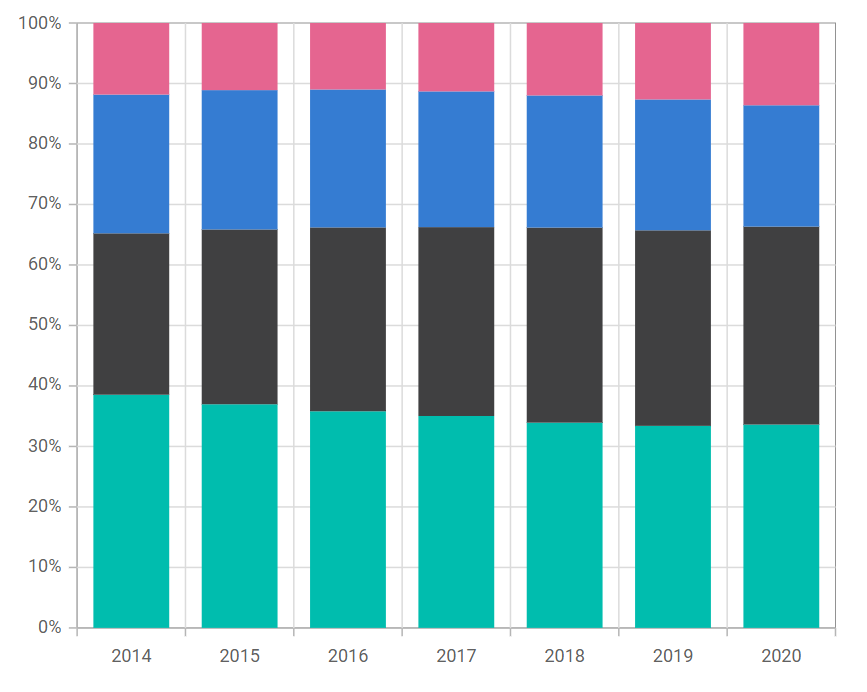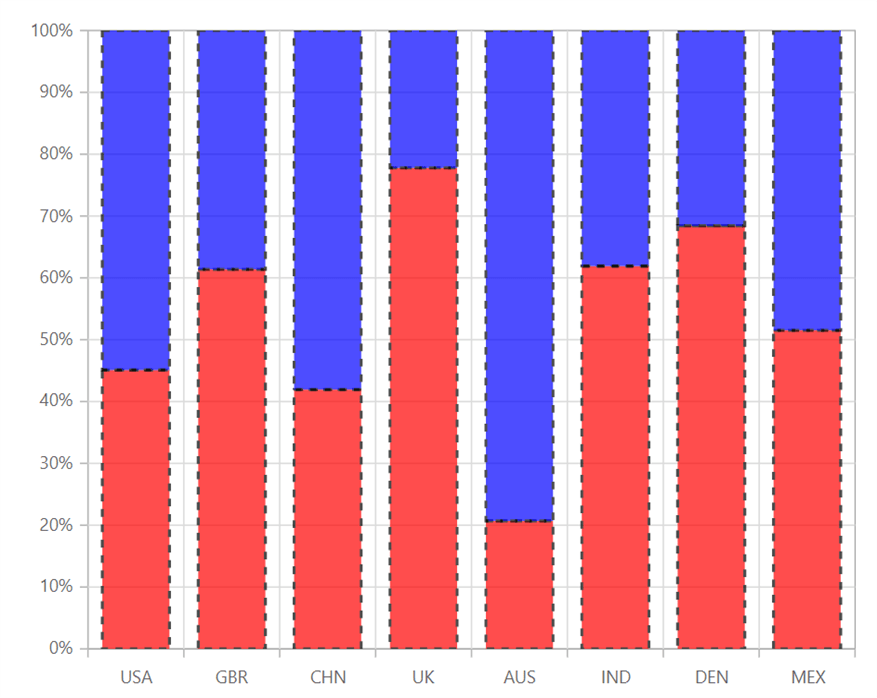# 100% Stacked Column in Blazor Charts Component

11 Aug 20234 minutes to read

## 100% Stacked Column

100% Stacked Column Chart displays multiple series of data as stacked columns, ensuring that the cumulative proportion of each stacked element always totals 100%. Hence, the y-axis will always be rendered with the range 0–100%. To render a 100% Stacked Column series, set the series Type as StackingColumn100.

``````@using Syncfusion.Blazor.Charts

<SfChart>
<ChartPrimaryXAxis ValueType="Syncfusion.Blazor.Charts.ValueType.Category"/>

<ChartSeriesCollection>
<ChartSeries DataSource="@MedalDetails" XName="X" YName="YValue" Type="ChartSeriesType.StackingColumn100">
</ChartSeries>
<ChartSeries DataSource="@MedalDetails" XName="X" YName="YValue1" Type="ChartSeriesType.StackingColumn100">
</ChartSeries>
</ChartSeriesCollection>
</SfChart>

@code{
public class ChartData
{
public string X { get; set; }
public double YValue { get; set; }
public double YValue1 { get; set; }
}

public List<ChartData> MedalDetails = new List<ChartData>
{
new ChartData { X= "USA", YValue= 46, YValue1=56 },
new ChartData { X= "GBR", YValue= 27, YValue1=17 },
new ChartData { X= "CHN", YValue= 26, YValue1=36 },
new ChartData { X= "UK", YValue= 56,  YValue1=16 },
new ChartData { X= "AUS", YValue= 12, YValue1=46 },
new ChartData { X= "IND", YValue= 26, YValue1=16 },
new ChartData { X= "DEN", YValue= 26, YValue1=12 },
new ChartData { X= "MEX", YValue= 34, YValue1=32},
};
}``````NOTE

Refer to our Blazor 100% Stacked Column Chart feature tour page to know about its other groundbreaking feature representations. Explore our Blazor 100% Stacked Column Chart Example to know how to render and configure the 100% Stacked Column type chart.

## Series customization

The following properties can be used to customize the 100% Stacked Column series.

``````@using Syncfusion.Blazor.Charts

<SfChart>
<ChartPrimaryXAxis ValueType="Syncfusion.Blazor.Charts.ValueType.Category"></ChartPrimaryXAxis>

<ChartSeriesCollection>
<ChartSeries DataSource="@DataSource" StackingGroup="Asia" XName="X" DashArray="5,5" Fill="red" Opacity="0.7" YName="YValue" Type="ChartSeriesType.StackingColumn100">
<ChartSeriesBorder Width="2" Color="black"></ChartSeriesBorder>
</ChartSeries>
<ChartSeries DataSource="@DataSource" StackingGroup="Asia" XName="X" DashArray="5,5" Fill="blue" Opacity="0.7" YName="YValue" Type="ChartSeriesType.StackingColumn100">
<ChartSeriesBorder Width="2" Color="black"></ChartSeriesBorder>
</ChartSeries>
</ChartSeriesCollection>
</SfChart>

@code{
public class ChartData
{
public string X { get; set; }
public double YValue { get; set; }
public double YValue1 { get; set; }
}

public List<ChartData> DataSource = new List<ChartData>
{
new ChartData { X= "USA", YValue= 46, YValue1=56 },
new ChartData { X= "GBR", YValue= 27, YValue1=17 },
new ChartData { X= "CHN", YValue= 26, YValue1=36 },
new ChartData { X= "UK", YValue= 56,  YValue1=16 },
new ChartData { X= "AUS", YValue= 12, YValue1=46 },
new ChartData { X= "IND", YValue= 26, YValue1=16 },
new ChartData { X= "DEN", YValue= 26, YValue1=12 },
new ChartData { X= "MEX", YValue= 34, YValue1=32},
};
}``````NOTE

Refer to our Blazor Charts feature tour page for its groundbreaking feature representations and also explore our Blazor Chart Example to know various chart types and how to represent time-dependent data, showing trends at equal intervals.